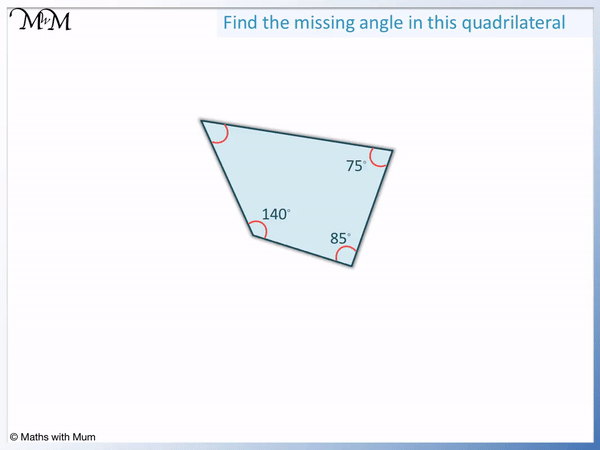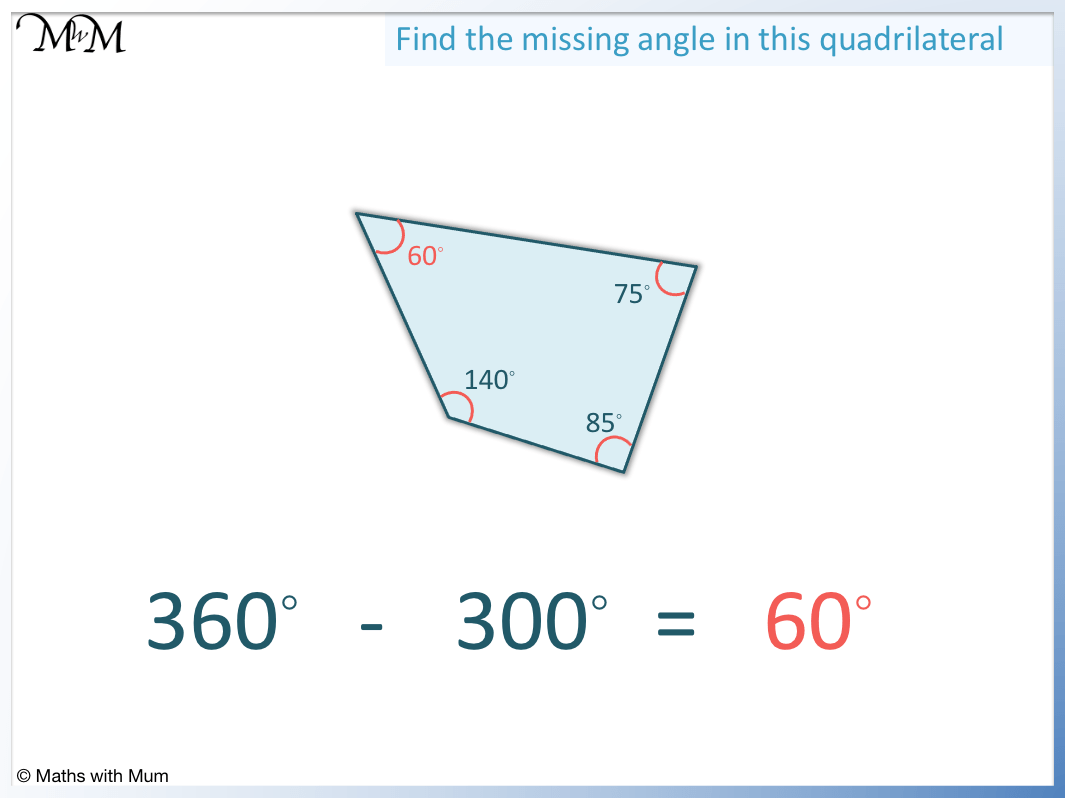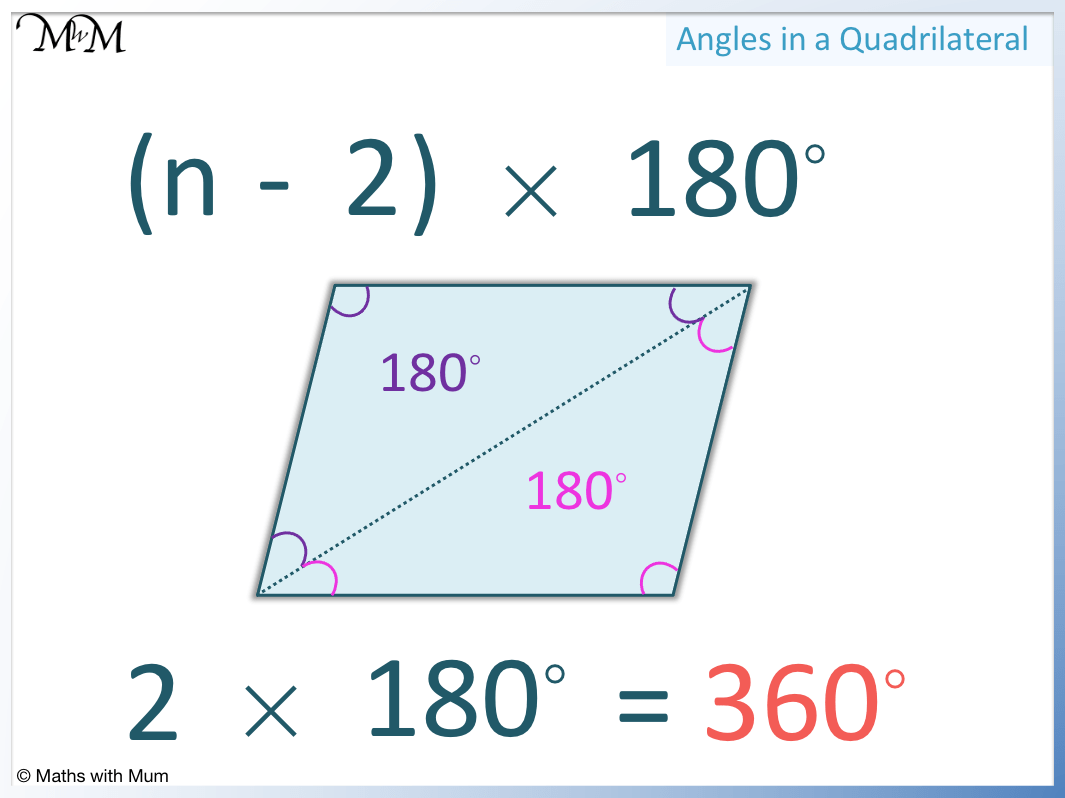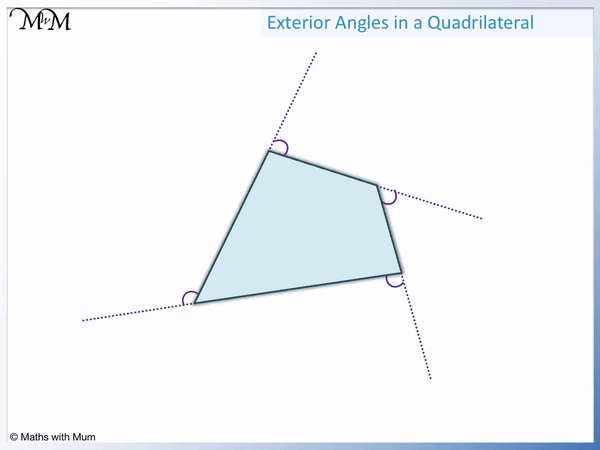• A quadrilateral is a 4-sided shape.
• This rule works because two triangles can be drawn inside the shapes. The angles in a triangle add to 180° and two lots of 180° is 360°.
• To find a missing angle in a quadrilateral, add up the 3 known angles and subtract this from 360°.
A quadrilateral has 4 sides and 4 angles. All 4 angles add up to 360°.• To find the missing angle, add up the 3 known angles and subtract this from 360°.
• 75° + 85° + 140° = 300°. The three known angles add to 300°.
• We subtract this from 360°. 360° – 300° = 60° and so the missing angle is 60°.Supporting LessonsThe four angles in a quadrilateral always add up to 360°. This rule is true for all quadrilaterals.

A quadrilateral is any 4-sided shape. Quadrilaterals also always have 4 angles.

For example, the 4 angles in a rectangle are all 90°. Four lots of 90° is 360°.Here are some more examples of quadrilaterals where the angles are shown adding up to 360°.## How to Find a Missing Angle in a Quadrilateral

The 4 angles in a quadrilateral always add up to 360°. To find a missing angle in a quadrilateral, add up the 3 known angles and subtract this result from 360°.

For example, here is a quadrilateral containing 3 known angles of 75°, 85° and 140°.The first step is to add up the 3 known angles. 75° + 85° + 140° = 300°.

The second step is to subtract this result from 360°.

360° – 300° = 60° and so, the missing angle is 60°.## How to Find Angles in a Parallelogram

If one angle in a parallelogram is known, all other angles can be calculated. To find missing angles in a parallelogram, use the following rules:

• Opposite angles are equal.

In the example below, one angle is known to be 120°.The angle opposite to this angle is the same size and so, angle a = 120°.

Angles b and c are both next to the 120° angle.

Therefore 120° + b = 180° and angle b = 60°.

120° + c = 180° and angle c = 60°.

b and c are opposite to each other, so we can see that they too are equal.We can check the angles in our parallelogram by making sure that all 4 angles add to 360°.

120° + 120° + 60° + 60° = 360° and so, the result is correct.

## Sum of Angles in a Quadrilateral Formula

The formula for the sum of the angles in a polygon is (n-2) × 180°, where n is the number of sides. A quadrilateral has 4 sides and so, n = 4. The formula (n-2) × 180° becomes 2 × 180° = 360°. Therefore the sum of the angles in a quadrilateral is 360°.

The formula for the sum of the angles in any polygon is (n-2) × 180°.The (n-2) part of the formula tells us how many triangles can be drawn inside any polygon.

n is the number of sides the shape has. Every time a new side is added, a new triangle can be formed.

In a quadrilateral, we can only form 2 triangles. For a quadrilateral, n = 4 and so the (n-2) becomes 4 – 2 which equals 2.

We have 2 triangles, so 2 lots of 180°.

2 × 180° = 360° and so, all 4 angles in a quadrilateral add to 360°.

## Why do Angles in a Quadrilateral Sum to 360 Degrees?

Angles in a quadrilateral add to 360° because two triangles can be made inside any quadrilateral by drawing straight lines from one corner to the other corners. Each triangle contains 180° and so, two triangles contain 360°.

In the example below, we see the four-sided shape divided into two triangles.The 3 angles in each triangle add up to 180°.

The combination of both triangle angles makes the full angles of the quadrilateral.

2 lots of 180° is 360°.

Therefore the angles in a quadrilateral sum to 360°.

All quadrilaterals sum to 360° because we can always draw two triangles inside them by connecting two opposite corners.

## Exterior Angles of a Quadrilateral

The exterior angles of a quadrilateral always add up to 360°. If the quadrilateral is shrunk down to the size of a point, all that is left are the exterior angles around the outside of it. Angles around a point add to 360° and so, the exterior angles add to 360°.

In the image below, we can see the exterior angles of a quadrilateral marked.We can see that these exterior angles encircle a point completely.

Angles around a point add to 360° because 360° is a full turn.